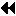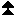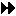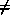Low-level topology functions

## Low-level topology functionsUp: Topology Constructors Next: An Application Example Previous: Partitioning of Cartesian structures

The two additional functions introduced in this section can be used to implement all other topology functions. In general they will not be called by the user directly, unless he or she is creating additional virtual topology capability other than that provided by MPI.

MPI_CART_MAP(comm, ndims, dims, periods, newrank)
[ IN comm] input communicator (handle)
[ IN ndims] number of dimensions of cartesian structure (integer)
[ IN dims] integer array of size ndims specifying the number of processes in each coordinate direction
[ IN periods] logical array of size ndims specifying the periodicity specification in each coordinate direction
[ OUT newrank] reordered rank of the calling process; MPI_UNDEFINED if calling process does not belong to grid (integer)

int MPI_Cart_map(MPI_Comm comm, int ndims, int *dims, int *periods, int *newrank)

MPI_CART_MAP(COMM, NDIMS, DIMS, PERIODS, NEWRANK, IERROR)
INTEGER COMM, NDIMS, DIMS(*), NEWRANK, IERROR
LOGICAL PERIODS(*)

MPI_CART_MAP computes an ``optimal'' placement for the calling process on the physical machine. A possible implementation of this function is to always return the rank of the calling process, that is, not to perform any reordering.

The function MPI_CART_CREATE(comm, ndims, dims, periods, reorder, comm_cart), with reorder = true can be implemented by calling MPI_CART_MAP(comm, ndims, dims, periods, newrank), then calling
MPI_COMM_SPLIT(comm, color, key, comm_cart), with color = 0 if newrankMPI_UNDEFINED
, color = MPI_UNDEFINED otherwise, and key = newrank.

The function MPI_CART_SUB(comm, remain_dims, comm_new) can be implemented by a call to MPI_COMM_SPLIT(comm, color, key, comm_new), using a single number encoding of the lost dimensions as color and a single number encoding of the preserved dimensions as key.

All other cartesian topology functions can be implemented locally, using the topology information that is cached with the communicator. ( End of advice to implementors.)
The corresponding new function for general graph structures is as follows.

MPI_GRAPH_MAP(comm, nnodes, index, edges, newrank)
[ IN comm] input communicator (handle)
[ IN nnodes] number of graph nodes (integer)
[ IN index] integer array specifying the graph structure, see
MPI_GRAPH_CREATE
[ IN edges] integer array specifying the graph structure
[ OUT newrank] reordered rank of the calling process; MPI_UNDEFINED if the calling process does not belong to graph (integer)

int MPI_Graph_map(MPI_Comm comm, int nnodes, int *index, int *edges, int *newrank)

MPI_GRAPH_MAP(COMM, NNODES, INDEX, EDGES, NEWRANK, IERROR)
INTEGER COMM, NNODES, INDEX(*), EDGES(*), NEWRANK, IERROR

The function MPI_GRAPH_CREATE(comm, nnodes, index, edges, reorder, comm_graph), with reorder = true can be implemented by calling MPI_GRAPH_MAP(comm, nnodes, index, edges, newrank), then calling
MPI_COMM_SPLIT(comm, color, key, comm_graph), with color = 0 if newrankMPI_UNDEFINED
, color = MPI_UNDEFINED otherwise, and key = newrank.

All other graph topology functions can be implemented locally, using the topology information that is cached with the communicator. ( End of advice to implementors.)Up: Topology Constructors Next: An Application Example Previous: Partitioning of Cartesian structures